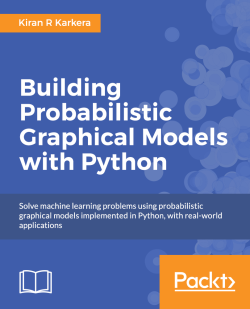### Building Probabilistic Graphical Models with Python4.3 (6 reviews total)
By Kiran R Karkera

• Constantly updated with 100+ new titles each month
• Breadth and depth in over 1,000+ technologies

With the increasing prominence in machine learning and data science applications, probabilistic graphical models are a new tool that machine learning users can use to discover and analyze structures in complex problems. The variety of tools and algorithms under the PGM framework extend to many domains such as natural language processing, speech processing, image processing, and disease diagnosis.

You've probably heard of graphical models before, and you're keen to try out new landscapes in the machine learning area. This book gives you enough background information to get started on graphical models, while keeping the math to a minimum.

Publication date:
June 2014
Publisher
Packt
Pages
172
ISBN
9781783289004

## Chapter 1. Probability

Before we embark on the journey through the land of graphical models, we must equip ourselves with some tools that will aid our understanding. We will first start with a tour of probability and its concepts such as random variables and the types of distributions.

We will then try to understand the types of questions that probability can help us answer and the multiple interpretations of probability. Finally, we will take a quick look at the Bayes rule, which helps us understand the relationships between probabilities, and also look at the accompanying concepts of conditional probabilities and the chain rule.

## The theory of probability

We often encounter situations where we have to exercise our subjective belief about an event's occurrence; for example, events such as weather or traffic that are inherently stochastic. Probability can also be understood as the degree of subjective belief.

When we talk about the weather (for example, this evening), it is understood that the weather can have multiple outcomes such as rainy, sunny, or cloudy. The space of all the possible outcomes is said to be an event (also called the sample space). For example, the outcomes of a throw of a dice would be a set of numbers from 1 to 6. While dealing with measurable outcomes such as the throw of a dice or today's weather (which can be rainy, sunny, or cloudy), we can assign a probability value to each outcome to encapsulate our degree of belief in those outcomes. An example of the notation used to express our belief is P(rainy)=0.3, which can be read as the probability of rain is 0.3 or 30 percent.

The axioms of probability that have been formulated by Kolmogorov are stated as follows:

• The probability of an event is a non-negative real number (that is, the probability that it will rain today may be small, but nevertheless will be greater than or equal to 0). This is explained in mathematical terms as follows: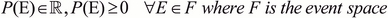• The probability of the occurrence of some event in the sample space is 1 (that is, if the weather events in our sample space are rainy, sunny, and cloudy, then one of these events has to occur), as shown in the following formula: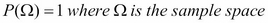• The sum of the probabilities of mutually exclusive events gives their union, as given in the following formula: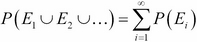When we discuss about the fairness (or unfairness) of a dice or a coin flip, we are discussing another key aspect of probability, that is, model parameters. The idea of a fair coin translates to the fact that the controlling parameter has a value of 0.5 in favor of heads, which also translates to the fact that we assume all the outcomes to be equally likely. Later in the book, we shall examine how many parameters are required to completely specify a probability distribution. However, we are getting ahead of ourselves. First let's learn about probability distribution.

A probability distribution consists of the probabilities associated with each measurable outcome. In the case of a discrete outcome (such as a throw of a dice or a coin flip), the distribution is specified by a probability mass function, and in the case of a continuous outcome (such as the height of students in a class), it is specified by a probability density function.

Let us see discrete distributions with an example. A coin flip has two outcomes: heads and tails, and a fair coin assigns equal probabilities to all outcomes. This means that the probability distribution is simple—for heads, it is 0.5 and for tails, it is 0.5. A distribution like this (for example, heads 0.3 and tails 0.7) would be the one that corresponds to a biased coin. The following graph shows the discrete probability distribution for the sum of values when two dice are thrown:A distribution that assigns equal probabilities to all outcomes is called a uniform distribution. This is one of the many distributions that we will explore.

Let's look at one of the common distributions associated with continuous outcomes, that is, the Gaussian or normal distribution, which is in the shape of a bell and hence called a bell curve (though there are other distributions whose shapes are similar to the bell shape). The following are some examples from the real world:

• Heights of students in a class are log-normally distributed (if we take the logarithm of the heights of students and plot it, the resulting distribution is normally distributed)

• Measurement errors in physical experiments

A Gaussian distribution has two parameters: mean (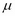) and variance (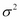). The parameters mean and variance determine the middle point and the dispersion of the distribution away from the mean, respectively.

The following graph shows multiple Gaussian distributions with different values of mean and variance. It can be seen that the more variance there is, the broader the distribution, whereas the value of the mean shifts the peak on the x axis, as shown in the following graph:## Goals of probabilistic inference

Now that we have understood the concept of probability, we must ask ourselves how this is used. The kind of questions that we ask fall into the following categories:

• The first question is parameter estimation, such as, is a coin biased or fair? And if biased, what is the value of the parameter?

• The second question is that given the parameters, what is the probability of the data? For example, what is the probability of five heads in a row if we flip a coin where the bias (or parameter) is known.

The preceding questions depend on the data (or lack of it). If we have a set of observations of a coin flip, we can estimate the controlling parameter (that is, parameter estimation). If we have an estimate of the parameter, we would like to estimate the probability of the data generated by the coin flips (the second question). Then, there are times when we go back and forth to improve the model.

• Is the model well-suited to the problem, is the third question that we may enquire about. Is there a single parameter that controls the results of the coin flipping experiment? When we wish to model a complicated phenomena (such as the traffic or weather prediction), there certainly exist several parameters in the model, where hundreds or even thousands of parameters are not unusual. In such cases, the question that we're trying to ask is, which model fits the data better? We shall see some examples in the later chapters on different aspects of model fit.

## Conditional probability

Let us use a concrete example, where we have a population of candidates who are applying for a job. One event (x) could be a set of all candidates who get an offer, whereas another event (y) could be the set of all highly experienced candidates. We might want to reason about the set of a conjoint event (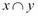), which is the set of experienced candidates who got an offer (the probability of a conjoint event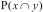is also written as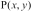). The question that raises is that if we know that one event has occurred, does it change the probability of occurrence of the other event. In this case, if we know for sure that a candidate got an offer, what does it tell us about their experience?

Conditional probability is formally defined as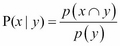, which can be read as the probability of x given that y occurred. The denominator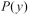is the sum of all possible outcomes of the joint distribution with the value of x summed out, that is,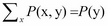.

## The chain rule

The chain rule allows us to calculate the joint distribution of a set of random variables using their conditional probabilities. In other words, the joint distribution is the product of individual conditional probabilities. Since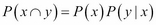, and if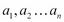are events,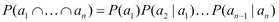.

We shall return to this in detail in graphical models, where the chain rule helps us decompose a big problem (computing the joint distribution) by splitting it into smaller problems (conditional probabilities).

## The Bayes rule

The Bayes rule is one of the foundations of the probability theory, and we won't go into much detail here. It follows from the definition of conditional probability, as shown in the following formula: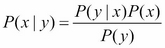From the formula, we can infer the following about the Bayes rule—we entertain prior beliefs about the problem we are reasoning about. This is simply called the prior term. When we start to see the data, our beliefs change, which gives rise to our final belief (called the posterior), as shown in the following formula: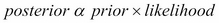Let us see the intuition behind the Bayes rule with an example. Amy and Carl are standing at a railway station waiting for a train. Amy has been catching the same train everyday for the past year, and it is Carl's first day at the station. What would be their prior beliefs about the train being on time?

Amy has been catching the train daily for the past year, and she has always seen the train arrive within two minutes of the scheduled departure time. Therefore, her strong belief is that the train will be at most two minutes late. Since it is Carl's first day, he has no idea about the train's punctuality. However, Carl has been traveling the world in the past year, and has been in places where trains are not known to be punctual. Therefore, he has a weak belief that the train could be even 30 minutes late.

On day one, the train arrives 5 minutes late. The effect this observation has on both Amy and Carl is different. Since Amy has a strong prior, her beliefs are modified a little bit to accept that the train can be as late as 5 minutes. Carl's beliefs now change in the direction that the trains here are rather punctual.

In other words, the posterior beliefs are influenced in multiple ways: when someone with a strong prior sees a few observations, their posterior belief does not change much as compared to their prior. On the other hand, when someone with a weak prior sees numerous observations (a strong likelihood), their posterior belief changes a lot and is influenced largely by the observations (likelihood) rather than their prior belief.

Let's look at a numerical example of the Bayes rule. D is the event that an athlete uses performance-enhancing drugs (PEDs). T is the event that the drug test returns positive. Throughout the discussion, we use the prime (') symbol to notate that the event didn't occur; for example, D' represents the event that the athlete didn't use PEDs.

P(D|T) is the probability that the athlete used PEDs given that the drug test returned positive. P(T|D) is the probability that the drug test returned positive given that the athlete used PEDs.

The lab doing the drug test claims that it can detect PEDs 90 percent of the time. We also learn that the false-positive rate (athletes whose tests are positive but did not use PEDs) is 15 percent, and that 10 percent of athletes use PEDs. What is the probability that an athlete uses PEDs if the drug test returned positive?

From the basic form of the Bayes rule, we can write the following formula: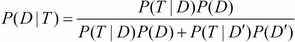Now, we have the following data:

• P(T|D): This is equal to 0.90

• P(T|D'): This is equal to 0.15 (the test that returns positive given that the athlete didn't use PEDs)

• P(D): This is equal to 0.1

When we substitute these values, we get the final value as 0.4, as shown in the following formula: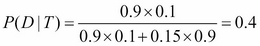This result seems a little counterintuitive in the sense that despite testing positive for PEDs, there's only a 40 percent chance that the athlete used PEDs. This is because the use of PEDs itself is quite low (only 10 percent of athletes use PEDs), and that the rate of false positives is relatively high (0.15 percent).

## Interpretations of probability

In the previous example, we noted how we have a prior belief and that the introduction of the observed data can change our beliefs. That viewpoint, however, is one of the multiple interpretations of probability.

The first one (which we have discussed already) is a Bayesian interpretation, which holds that probability is a degree of belief, and that the degree of belief changes before and after accounting for evidence.

The second view is called the Frequentist interpretation, where probability measures the proportion of outcomes and posits that the prior belief is an incorrect notion that is not backed up by data.

To illustrate this with an example, let's go back to the coin flipping experiment, where we wish to learn the bias of the coin. We run two experiments, where we flip the coin 10 times and 10000 times, respectively. In the first experiment, we get 7 heads and in the second experiment, we get 7000 heads.

From a Frequentist viewpoint, in both the experiments, the probability of getting heads is 0.7 (7/10 or 7000/10000). However, we can easily convince ourselves that we have a greater degree of belief in the outcome of the second experiment than that of the first experiment. This is because the first experiment's outcome has a Bayesian perspective that if we had a prior belief, the second experiment's observations would overwhelm the prior, which is unlikely in the first experiment.

For the discussion in the following sections, let us consider an example of a company that is interviewing candidates for a job. Prior to inviting the candidate for an interview, the candidate is screened based on the amount of experience that the candidate has as well as the GPA score that the candidate received in his graduation results. If the candidate passes the screening, he is called for an interview. Once the candidate has been interviewed, the company may make the candidate a job offer (which is based on the candidate's performance in the interview). The candidate is also evaluating going for a postgraduate degree, and the candidate's admission to a postgraduate degree course of his choice depends on his grades in the bachelor's degree. The following diagram is a visual representation of our understanding of the relationships between the factors that affect the job selection (and postgraduate degree admission) criteria: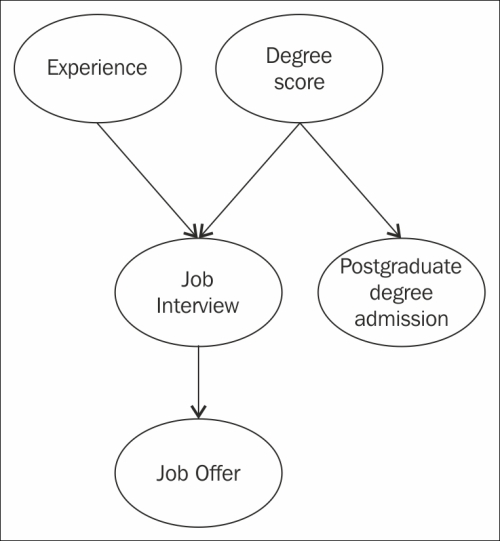## Random variables

The classical notion of a random variable is the one whose value is subject to variations due to chance (Wikipedia). Most programmers have encountered random numbers from standard libraries in programming languages. From a programmer's perspective, unlike normal variables, a random variable returns a new value every time its value is read, where the value of the variable could be the result of a new invocation of a random number generator.

We have seen the concept of events earlier, and how we could consider the probability of a single event occurring out of the set of measurable events. It may be suitable, however, to consider the attributes of an outcome.

In the candidate's job search example, one of the attributes of a candidate is his experience. This attribute can take multiple values such as highly relevant or not relevant. The formal machinery for discussing attributes and their values in different outcomes is called random variables [Koller et al, 2.1.3.1].

Random variables can take on categorical values (such as {Heads, Tails} for the outcomes of a coin flip) or real values (such as the heights of students in a class).

## Marginal distribution

We have seen that the job hunt example (described in the previous diagram) has five random variables. They are grades, experience, interview, offer, and admission. These random variables have a corresponding set of events.

Now, let us consider a subset X of random variables, where X contains only the Experience random variable. This subset contains the events highly relevant and not relevant.

If we were to enlist the probabilities of all the events in the subset X, it would be called a marginal distribution, an example of which can be found in the following formula: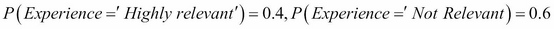Like all valid distributions, the probabilities should sum up to 1.

The set of random variables (over which the marginal distribution is described) can contain just one variable (as in the previous example), or it could contain several variables, such as {Experience, Grades, Interview}.

## Joint distribution

We have seen that the marginal distribution is a distribution that describes a subset of random variables. Next, we will discuss a distribution that describes all the random variables in the set. This is called a joint distribution. Let us look at the joint distribution that involves the Degree score and Experience random variables in the job hunt example:

 Degree score Relevant Experience Highly relevant Not relevant Poor 0.1 0.1 0.2 Average 0.1 0.4 0.5 Excellent 0.2 0.1 0.3 0.4 0.6 1

The values within the dark gray-colored cells are of the joint distribution, and the values in the light gray-colored cells are of the marginal distribution (sometimes called that because they are written on the margins). It can be observed that the individual marginal distributions sum up to 1, just like a normal probability distribution.

Once the joint distribution is described, the marginal distribution can be found by summing up individual rows or columns. In the preceding table, if we sum up the columns, the first column gives us the probability for Highly relevant, and the second column for Not relevant. It can be seen that a similar tactic applied on the rows gives us the probabilities for degree scores.

## Independence

The concept of independent events can be understood by looking at an example. Suppose we have two dice, and when we roll them together, we get a score of 2 on one die and a score of 3 on the other. It is not difficult to see that the two events are independent of each other because the outcome of a roll of each dice does not influence or interact with the other one.

We can define the concept of independence in multiple ways. Assume that we have two events a and b and the probability of the conjunction of both events is simply the product of their probabilities, as shown in the following formula: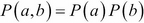If we were to write the probability of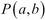as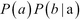(that is, the product of probability of a and probability of b given that the event a has happened), if the events a and b are independent, they resolve to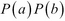.

An alternate way of specifying independence is by saying that the probability of a given b is simply the probability of a, that is, the occurrence of b does not affect the probability of a, as shown in the following formula: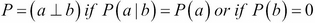It can be seen that the independence is symmetric, that is,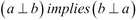. Although this definition of independence is in the context of events, the same concept can be generalized to the independence of random variables.

## Conditional independence

Given two events, it is not always obvious to determine whether they are independent or not. Consider a job applicant who applied for a job at two companies, Facebook and Google. It could result in two events, the first being that a candidate gets an interview call from Google, and another event that he gets an interview call from Facebook. Does knowing the outcome of the first event tell us anything about the probability of the second event? Yes, it does, because we can reason that if a candidate is smart enough to get a call from Google, he is a promising candidate, and that the probability of a call from Facebook is quite high.

What has been established so far is that both events are not independent. Supposing we learn that the companies decide to send an interview invite based on the candidate's grades, and we learn that the candidate has an A grade, from which we infer that the candidate is fairly intelligent. We can reason that since the candidate is fairly smart, knowing that the candidate got an interview call from Google does not tell us anything more about his perceived intelligence, and that it doesn't change the probability of the interview call from Facebook. This can be formally annotated as the probability of a Facebook interview call, given a Google interview call AND grade A, is equal to the probability of a Facebook interview call given grade A, as shown in the following formula: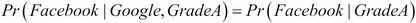In other words, we are saying that the invite from Facebook is conditionally independent of the invite from Google, given the candidate has grade A.

## Types of queries

Having learned about joint and conditional probability distributions, let us turn our attention to the types of queries we can pose to these distributions.

### Probability queries

This is the most common type of query, and it consists of the following two parts:

• The evidence: This is a subset E of random variables which have been observed

• The query: This is a subset Y of random variables

We wish to compute the value of the probability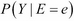, which is the posterior probability or the marginal probability over Y. Using the job seeker example again, we can compute the marginal distribution over an interview call, conditioned on the fact that Degree score = Grade A.

### MAP queries

Maximum a posteriori (MAP) is the highest probability joint assignment to some subsets of variables. In the case of the probability query, it is the value of the probability that matters. In the case of MAP, calculating the exact probability value of the joint assignment is secondary as compared to the task of finding the joint assignment to all the random variables.

It is possible to return multiple joint assignments if the probability values are equal. We shall see from an example that in the case of the joint assignment, it is possible that the highest probability from each marginal value may not be the highest joint assignment (the following example is from Koller et al).

Consider two non-independent random variables X and Y, where Y is dependent on X. The following table shows the probability distribution over X:

X0

X1

0.4

0.6

We can see that the MAP assignment for the random variable X is X1 since it has a higher value. The following table shows the marginal distribution over X and Y:

P(Y|X)

Y0

Y1

X0

0.1

0.9

X1

0.5

0.5

The joint distribution over X and Y is listed in the following table:

Assignment

Value

X0, Y0

0.04

X0, Y1

0.36

X1, Y0

0.3

X1, Y1

0.3

In the joint distribution shown in the preceding table, the MAP assignment to random variables (X, Y) is (X0, Y1), and that the MAP assignment to X (X1) is not a part of the MAP of the joint assignment. To sum up, the MAP assignment cannot be obtained by simply taking the maximum probability value in the marginal distribution for each random variable.

A different type of MAP query is a marginal MAP query where we only have a subset of the variables that forms the query, as opposed to the joint distribution. In the previous example, a marginal MAP query would be MAP (Y), which is the maximum value of the MAP assignment to the random variable Y, which can be read by looking at the joint distribution and summing out the values of X. From the following table, we can read the maximum value and determine that the MAP (Y) is Y1:

Assignment

Value

Y0

0.34

Y1

0.66

### Note

The data for the marginal query has been obtained from Querying Joint Probability Distributions by Sargur Srihari. You can find it at http://www.cedar.buffalo.edu/~srihari/CSE574/Chap8/Ch8-PGM-Directed/8.1.2-QueryingProbabilityDistributions.pdf.

## Summary

In this chapter, we looked at the concepts of basic probability, random variables, and the Bayes theorem. We also learned about the chain rule and joint and marginal distributions with the use of a candidate job search example, which we shall return to in the later chapters. Having obtained a good grasp on these topics, we can now move on to exploring Bayes and Markov networks in the forthcoming chapters, where we will formally describe these networks to answer some of the probability queries we discussed in this chapter. While this chapter was completely theoretical, from the next chapter, we shall implement the Python code to seek answers to our questions.